### States of Matter - Gases and Liquids - Solutions

CBSE Class–12 Subject Chemistry

NCERT Solutions
Chapter – 01
The Solid State

1. Define the term 'amorphous'. Give a few examples of amorphous solids.

Ans. Amorphous solids are the solids whose constituent particles are irreguraly arranged and have short range order. These solids are isotropic in nature and melt over a range of temperature. Therefore, amorphous solids are sometimes called pseudo solids or super cooled liquids. They do not have definite heat of fusion. When cut with a sharp-edged tool, they cut into two pieces with irregular surfaces. Examples of amorphous solids include glass, rubber, and plastic.

2. What makes a glass different from a solid such as quartz? Under what conditions could quartz be converted into glass?

Ans. The arrangement of the constituent particles makes glass different from quartz. In glass, the constituent particles have short-range order, but in quartz, the constituent particles have long range order.

Quartz can be converted into glass by heating and then cooling it rapidly.

3. Classify the following as amorphous or crystalline solids:

Polyurethane, naphthalene, benzoic acid, teflon, potassium nitrate, cellophane, polyvinyl chloride, fibre glass, copper.

Ans. Amorphous solids: Polyurethane, teflon, cellophane, polyvinyl chloride, fibre glass

Crystalline solids: Naphthalene, benzoic acid, potassium nitrate, copper

4. (i) What is meant by the term 'coordination number'?

(ii) What is the coordination number of atoms:

(a) in a cubic close-packed structure?

(b) in a body-centred cubic structure?

Ans. (i) The number of nearest neighbours of any constituent particle present in the crystal lattice is called its coordination number.

(ii) The coordination number of atoms

(a) in a cubic close-packed structure is 12, and

(b) in a body-centred cubic structure is 8

5. How can you determine the atomic mass of an unknown metal if you know its density and the dimension of its unit cell? Explain.

Ans. By knowing the density of an unknown metal and the dimension of its unit cell, the atomic mass of the metal can be determined.

Let ‘a’ be the edge length of a unit cell of a crystal, ‘d’ be the density of the metal, ‘m’ be the  atomic mass of one atom of the metal and ‘z’ be the number of atoms in the unit cell.

Now, density of the unit cell =Mass of the unit cell/ Volume of the unit cell

d= z x m / a x NA……………………(i)

[Since mass of the unit cell = Number of atoms in the unit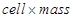of one atom

and mass of one atom = atomic mass / NA]

[Volume of the unit cell = (Edge length of the cubic unit cell)3]

From equation (i), we have :

m = d x a3 x NA / z……………………..(ii)

if it is not a cubic unit cell then dimensions of unit cell will be a, b, c and volume of unit cell = axbxc

so m= d x (abc) x N/ z

6. 'Stability of a crystal is reflected in the magnitude of its melting point'. Comment. Collect melting points of solid water, ethyl alcohol, diethyl ether and methane from a data book. What can you say about the intermolecular forces between these molecules?

Ans. Higher the melting point, greater is the intermolecular force of attraction and greater is the stability. A substance with higher melting point is more stable than a substance with lower melting point.

The melting points of the given substances are:

Solid water = 273 K

Ethyl alcohol = 158.8 K

Diethyl ether = 156.85 K

Methane = 89.34 K

Now, on observing the values of the melting points, it can be said that among the given substances, the intermolecular force in solid water is the strongest and that in methane is the weakest. This is becase of existance of hydrogen bonding ( strong interaction ) in solid water (ice) and vanderwall forces which are weaker force exist  in methane.

7. How will you distinguish between the following pairs of terms:

(i) Hexagonal close-packing and cubic close-packing?

(ii) Crystal lattice and unit cell?

(iii) Tetrahedral void and octahedral void?

Ans. A 2-D hexagonal close-packing contains two types of triangular voids (shaded a and b) as shown in figure 1. Let us call this 2-D structure as layer A. Now, particles are kept in the voids present in layer A (it can be easily observed from figures 2 and 3 that only one of the voids will be occupied in the process, i.e., either a or b). Let us call the particles or spheres present in the voids of layer A as layer B. Now, two types of voids are present in layer B (c and d). Unlike the voids present in layer A, the two types of voids present in layer B are not similar. Void c is surrounded by 4 spheres and is called the tetrahedral void. Void d is surrounded by 6 spheres and is called the octahedral void.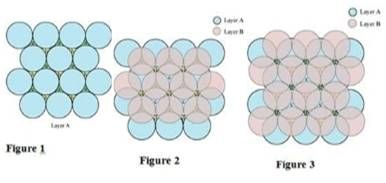Now, the next layer can be placed over layer B in 2 ways.

Case 1: When the third layer (layer C) is placed over the second one (layer B) in such a manner that the spheres of layer C occupy the tetrahedral voids c.

In this case we get hexagonal close-packing. This is shown in figure 4. In figure 4.1, layer B is present over the voids a and layer C is present over the voids c. In figure 4.2, layer B is present over the voids b and layer C is present over the voids c. It can be observed from the figure that in this arrangement, the spheres present in layer C are present directly above the spheres of layer A. Hence, we can say that the layers in hexagonal close-packing are arranged in an ABAB…..pattern.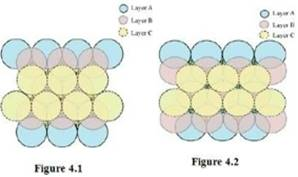Case 2: When the third layer (layer C) is placed over layer B in such a manner that the spheres of layer C occupy the octahedral voids d.

In this case we get cubic close-packing. In figure 5.1, layer B is present over the voids a and layer C is present over the voids d. In figure 5.2, layer B is present over the voids b and layer C is present over the voids d. It can be observed from the figure that the arrangement of particles in layer C is completely different from that in layers A or B. When the fourth layer is kept over the third layer, the arrangement of particles in this layer is similar to that in layer A. Hence, we can say that the layers in cubic close-packing are arranged in an ABCABC…..pattern.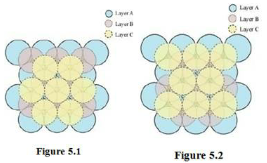8. How many lattice points are there in one unit cell of each of the following lattice?

(i) Face-centred cubic
(ii) Face-centred tetragonal
(iii) Body-centred

Ans. (i) There are 14 (8 from the corners + 6 from the faces) lattice points in face-centred cubic.
(ii) There are 14 (8 from the corners + 6 from the faces) lattice points in face-centred tetragonal.
(iii) There are 9 (1 from the centre + 8 from the corners) lattice points in body-centred cubic.

9. Explain

(i) The basis of similarities and differences between metallic and ionic crystals.
(ii) Ionic solids are hard and brittle.

Ans. (i) The basis of similarities between metallic and ionic crystals is that both these crystal types are held by the electrostatic force of attraction. In metallic crystals, the electrostatic force acts between the positive metal ions (Kernels) and the electrons. In ionic crystals, it acts between the oppositely-charged ions (cation and anion). Hence, both have high melting points.

The basis of differences between metallic and ionic crystals is that in metallic crystals, the electrons are free to move and so, metallic crystals can conduct electricity. However, in ionic crystals, the ions are not free to move. As a result, they cannot conduct electricity. However, in molten state or in aqueous solution, they do conduct electricity.

(ii) The constituent particles of ionic crystals are ions. These ions are held together in three-dimensional arrangements by the electrostatic force of attraction. Since the electrostatic force of attraction is very strong, the charged ions are held in fixed positions. This is the reason why ionic crystals are hard and brittle.

10. Calculate the efficiency of packing in case of a metal crystal for

(i) simple cubic
(ii) body-centred cubic
(iii) face-centred cubic (with the assumptions that atoms are touching each other).

Ans. (i)Simple cubic

In a simple cubic lattice, the particles are located only at the corners of the cube and touch each other along the edge.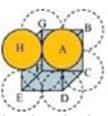Let the edge length of the cube be ‘a’ and the radius of each particle be r.

So, we can write: = 2r

Now, volume of the cubic unit cell =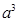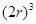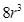We know that the number of particles per unit cell is 1.

Therefore, volume of the occupied by atoms in unit cell = 4/3 x 3.14 x r 3

Hence, packing efficiency =  (volume occupied by particles in unit cell / volume of cubic unit cell) x 100

= (4/3 x 3.14 x r3 / a3 ) x 100

and a= 2r

=52.4%

(ii) Body-centred cubic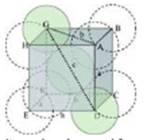It can be observed from the above figure that the atom at the centre is in contact with the other two atoms diagonally arranged.

From ΔFED, we have:

b2=a2+a2⇒b2=2a2⇒b=1.414a

Again, from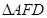, we have:

c2=a2+b2⇒c2=a2+2a2⇒c2=3a2 (Since b2=2a2)

c =1.73 a

Let the radius of the atom be r.

Length of the body diagonal, c= 4r

= 1.73 a = 4r

Volume of the cube,  a3 = (4r/1.73)3

A body-centred cubic lattice contains 2 atoms.

So, volume of the occupied by atoms in cubic lattice =  2 x 4/3 x 3.14 x r3

Therefore, Packing efficiency = Volume occupied by two spheres in the unit cell / Total volume of the unit cell x 100

= (2 x 4/3 x 3.14 x r3 ) / a3 x 100

and a3 = (4r/1.73)3

packing effiency = 68 %

(iii) Face-centred cubic

Let the edge length of the unit cell be ‘a’ and the length of the face diagonal AC be b.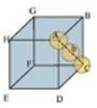From ΔABC, we have: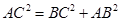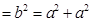b = 1.414a

Let r be the radius of the atom.
Now, from the figure,it can be observed that:
b=4r
Now, volume of the cube, a3
We know that the number of atom per unit cell is 4.
So, volume of the occupied by atoms of unit cell= 4 x 4/3 x 3.14 x r3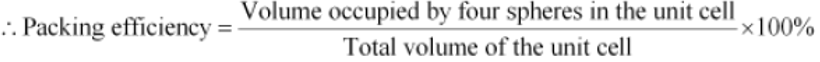Packing efficiency = ( 4 x 4/3 x3.14 x r3 ) / a3 x 100

and 1.414a = 4r

= 74%